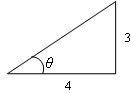# Tangent function

To use this function, choose Calc > Calculator.

The tangent of an angle is the ratio of its sine to its cosine. For an acute angle of a right triangle, this is equivalent to the ratio of the length of the opposite leg to the length of the adjacent leg, measured in radiansThe tangent of angle θ is the ratio 3/4, or 0.75.

The tangent can be calculated for any angle θ on the unit circle measured from the origin (counterclockwise from the positive x-axis), except at angles where the sine equals 0 (e.g., π/2, -π/2 ).